5心关爱护航 东南A5翼舞西安上市发布

11月25日，一场主题为“绚舞东南 翼家之选”的东南A5翼舞新车上市发布会，于陕西汽贸东南汽车4s店成功举办，现场一众媒体、嘉宾与东南人员共同见证了发布会的盛况，率先体验东南A5翼舞的品质之美。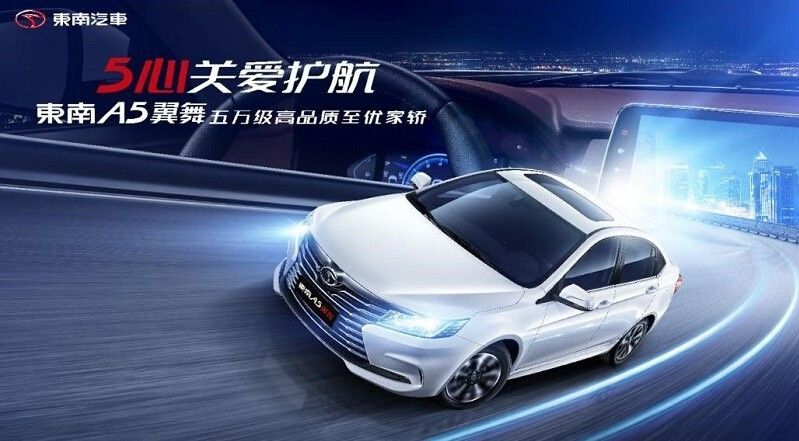自11月16日在广州车展上闪耀登场以来，东南翼舞即凭借更时尚、更舒适、更智能、更高品质等优势赚足消费者的眼球，同时新车售价仅为4.99-7.59万元，超高的性价比让人更为期待。此次“绚舞东南 翼家之选”上市发布会，不仅大大满足了众多粉丝想要第一时间近距离体验新车的心愿，而且现场公布的多重促销优惠也让到场消费者惊喜，下面就让小编带大家来回顾一下现场发布会的活动盛况吧！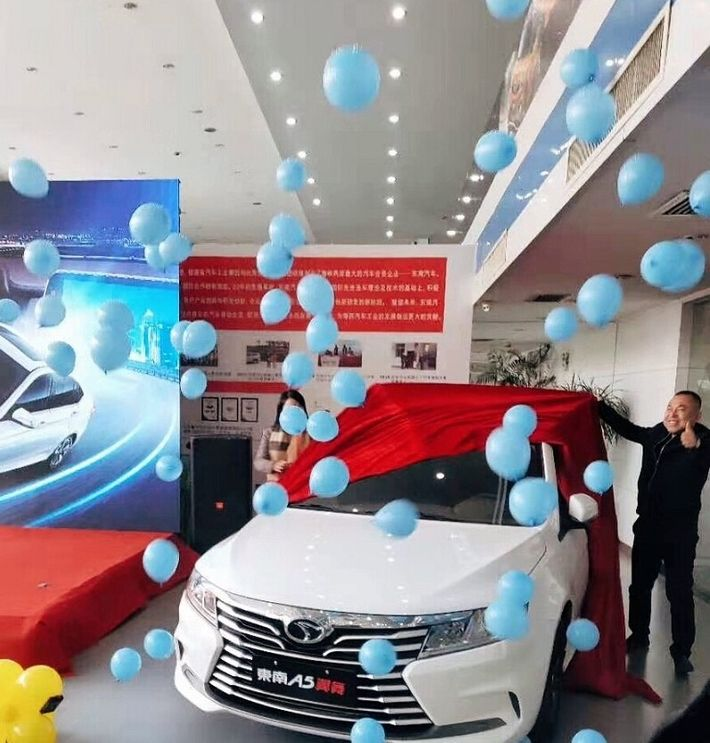互动签到，惊喜不断

活动当天吸引了多家媒体和众多车友到场参与，现场人头汹涌。发布会尚未开始前，来宾们纷纷参与主办方精心安排的线上创意签到，签到成功后还能通过手机摇一摇参与互动小游戏赢取礼品，气氛十分活跃。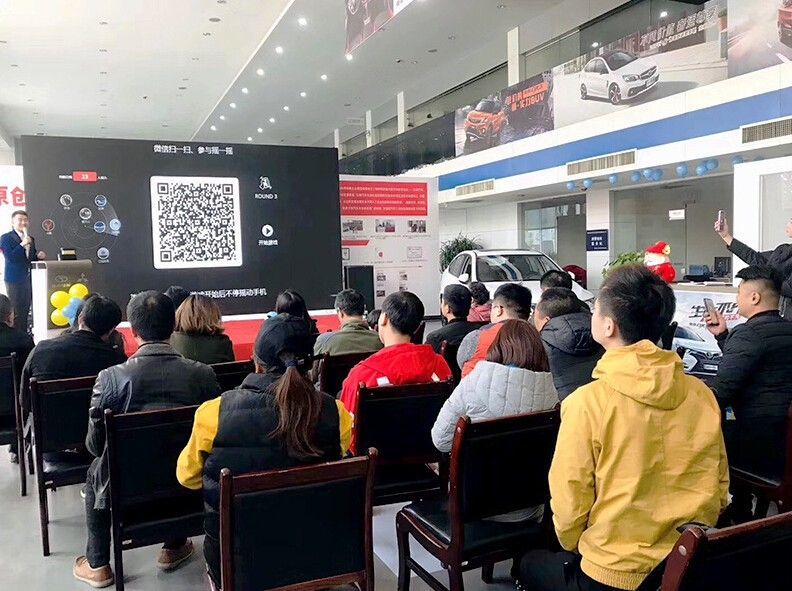精彩的暖场歌舞表演过后，现场领导、嘉宾以及选定的家庭代表一同上台，共同启动了发布会的开幕装置并为新车揭幕——东南翼舞在大家殷切期盼的目光中惊艳亮相，旋即把现场的气氛推向了高潮！

售价、促销政策公布，引人心动

东南翼舞的亮相让到场观众兴奋不已，随后现场领导公布的新车售价以及一系列新车促销政策更让消费者砰然心动。不到5万元的起售价加上三重惊喜大礼，着实又为东南翼舞吸了一波粉。

二重惊喜大礼：

金融礼：享受24期0利息；

质保礼：享受5年或15万公里质保；

六位一体绕车讲解，深入彰显新车魅力

为了让现场客户对东南翼舞的产品优势有更深入的了解，现场主持和领导更对新车进行六位一体绕车讲解，并结合新车“五心关爱护航”的传播核心进行了重点介绍，专业又不乏趣味的讲解让现场车友听得热情高涨。

作为东南汽车在轿车领域的最新力作，东南翼舞继承了东南家族翼立方的原创设计基因，鹰击式前脸如雄鹰展翅般霸气十足，鹰眼式LED前大灯组炯炯有神，流畅的车身线条营造强烈的运动气息。内饰上，悬浮式设计的中控台显得十分时尚，豪华皮质座椅不仅让整车内饰更显档次，也为消费者提供了更舒适的乘坐体验。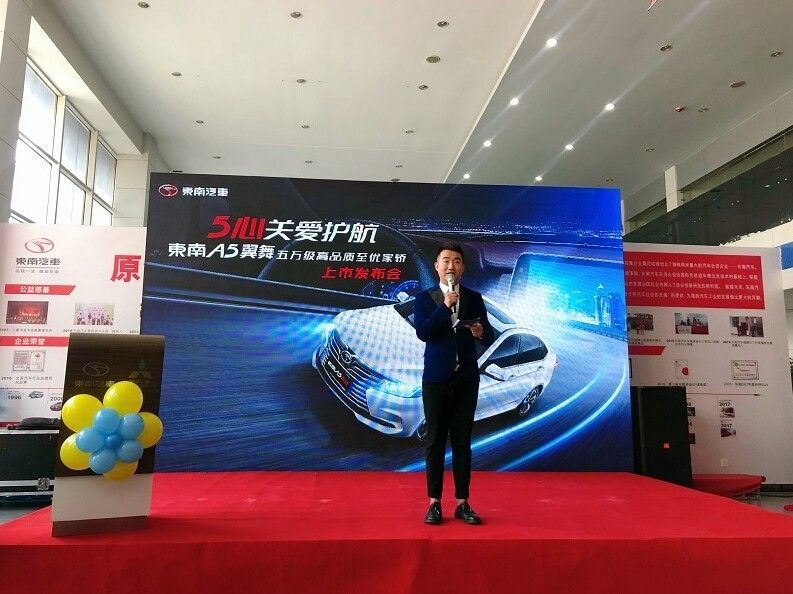空间方面，东南翼舞长宽高分别为4588/1780/1485mm，轴距达到2625mm，超越同级的驾乘空间表现出色。现场观众纷纷坐进车内体验，对宽大的车内空间赞不绝口。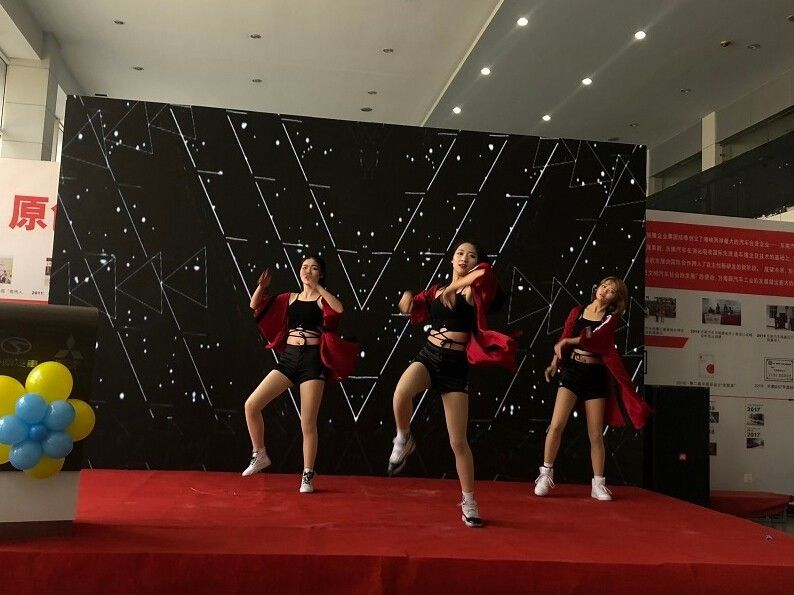高效动力，用得省心

史上最严国六政策即将来袭，众多消费者正为此犯愁，东南汽车贴心地考虑到这一点。东南翼舞是同级首款搭载满足国六排放标准动力系统的车型，搭载三菱全铝发动机，匹配Punch智能CVT无级变速器，动力高效、稳定，同时兼具出色的燃油经济性，让消费者日常用车更省心。不仅动力高效，东南翼舞还搭载同级罕见的麦弗逊式独立前悬架/多连杆式独立后悬架，保证加速和制动时的平顺性、舒适性以及行驶稳定性。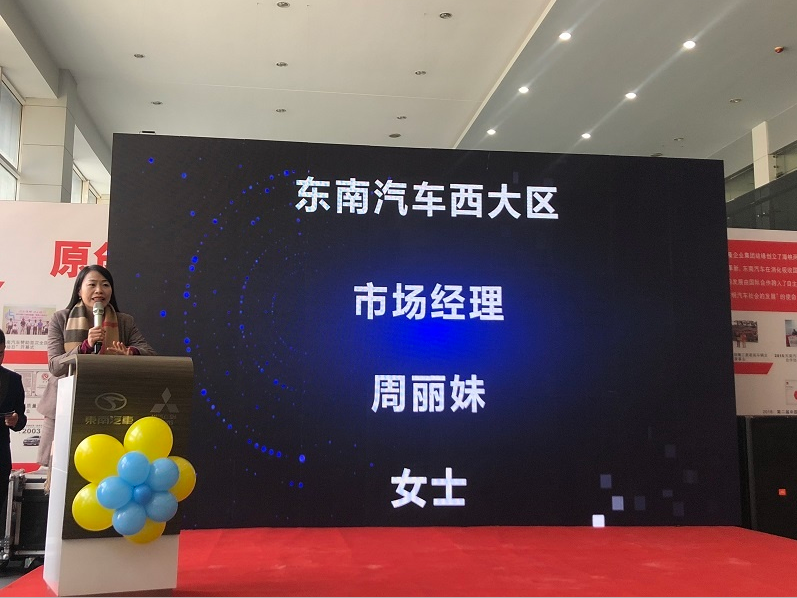实力安全，驾控安心

而在大家普遍关注的汽车安全方面，东南翼舞的表现也相当优秀。新车采用高刚性安全车身，配备正/副驾驶座双安全气囊、高清倒车影像系统以及车后侧高敏度倒车雷达等多项安全配置，国六车型更搭载ESC车身动态稳定系统、ESS刹车警示系统、HAC上坡辅助系统、TPMS(直接式胎压监测系统)等丰富的主动安全配置，全方位多重保障出行安全；智慧科技，买的舒心

作为定位“五万级高品质至优家轿”的车型，东南翼舞在配置方面的表现足够厚道。9寸中控大屏吸睛无数，配备的智能导航系统、车载WIFI车联网系统、车载蓝牙系统以及百度Carlife等功能让整车的科技感提升不少，加上智能无钥匙进入、脚踢尾门感应系统及一键启动系统等多项越级智能配置应有尽有，给予车主及其家人满满的舒适享受！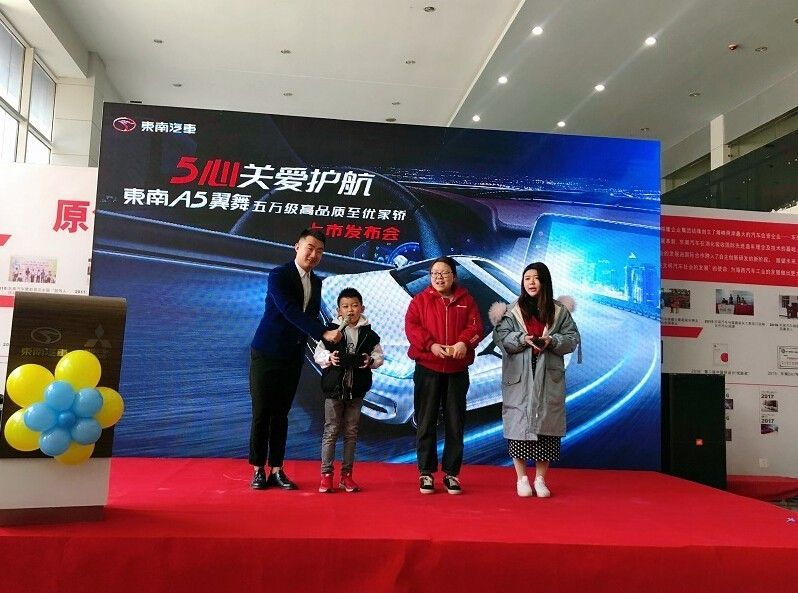严苛品质，开的放心

23年来东南汽车始终坚持匠心品质的造车态度，每款车型都是经过严苛测试打造而来，东南翼舞也不例外。凭借着东南精湛的制造工艺以及上市前一系列耐久试验、涉水池试验、冷暖房试验以及高温、高寒、高原“三高测试”的反复调校，东南翼舞的品质绝对值得信赖！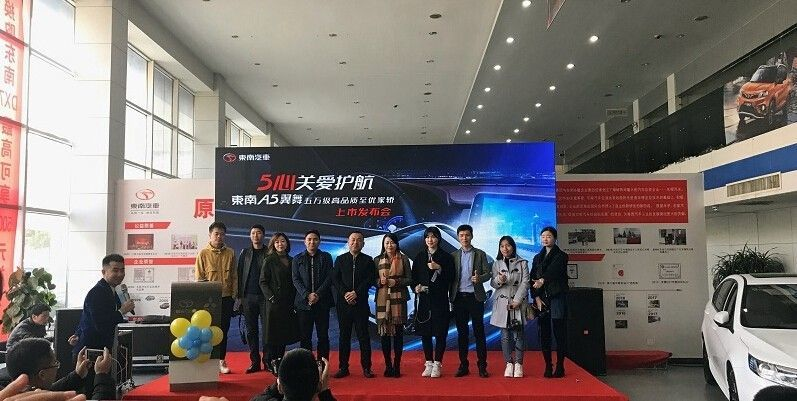至此，东南A5翼舞新车上市发布会取得圆满成功！相信东南翼舞将会更好地满足家庭消费者多元化的用车需求，同时也为正奋斗打拼的“新时代青年”提供更舒适、更高品质的出行体验。

|分享

•已阅！握爪
•我手滑为你点赞
•128个赞！
•不明觉厉
•阅后既醉
•有钱！任性
•照片太美，我不敢看
•干货！杠杠的
•高大上
•膜拜中！
•请接受我的膝盖
•猫哥/猫妹么么哒
•神吐槽
•我只笑笑不说话
•我想静静
•窒息
##### 资讯相关车系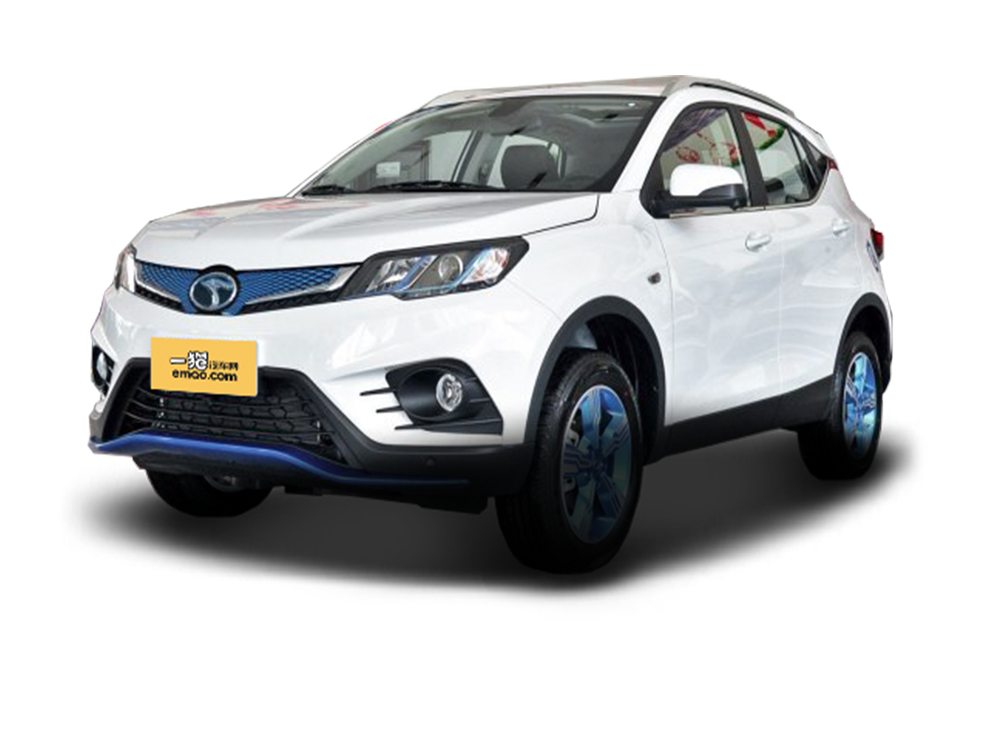##### 热度排行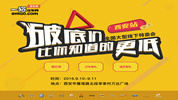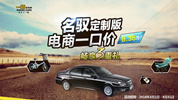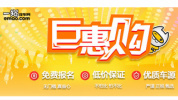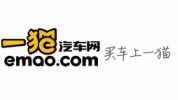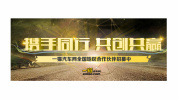• 阿斯顿·马丁
• 奥迪
• 阿尔法罗密欧
• ALPINA

• 巴博斯
• 宝骏
• 宝马
• 保时捷
• 北汽制造
• 奔驰
• 奔腾
• 本田
• 比亚迪
• 标致
• 别克
• 宾利
• 布加迪
• 北汽威旺
• 北京
• 北汽绅宝
• 北汽幻速
• 北汽新能源
• 宝沃
• 比速汽车
• 北汽道达

• 昌河
• 长安
• 长城
• 长安商用
• 成功汽车
• 长江EV
• 长安轻车型

• 大众
• 道奇
• 东风
• 东风风神
• 东风小康
• 东南
• DS
• 东风风行
• 东风风度
• 东风风光

• 法拉利
• 菲亚特
• 丰田
• 福迪
• 福特
• 福田汽车
• 福汽启腾
• 风诺

• GMC
• 广汽传祺
• 广汽吉奥
• 观致

• 哈飞
• 海格
• 海马
• 华泰
• 黄海
• 恒天
• 红旗
• 哈弗
• 华颂
• 华凯
• 华泰新能源
• 汉腾汽车

• Jeep
• 江淮
• 江铃
• 捷豹
• 金杯
• 九龙
• 吉利汽车
• 金旅
• 金龙
• 江铃集团轻汽
• 江铃集团新能源
• 君马汽车
• 捷途

• 开瑞
• 凯迪拉克
• 科尼赛克
• 克莱斯勒
• KTM
• 卡威
• 凯翼
• 康迪
• 康迪电动汽车集团

• 猎豹汽车
• 兰博基尼
• 劳斯莱斯
• 雷克萨斯
• 雷诺
• 理念
• 力帆
• 莲花汽车
• 林肯
• 铃木
• 陆风
• 路虎
• 路特斯
• 领志
• 领克
• 零跑汽车

• MG
• MINI
• 马自达
• 玛莎拉蒂
• 迈凯伦
• 摩根

• 纳智捷

• 讴歌
• 欧宝
• 欧朗
• 欧拉
• 欧尚汽车

• 帕加尼

• 奇瑞
• 启辰
• 起亚
• 前途
• 庆铃汽车

• 日产
• 荣威
• 瑞麒汽车
• 如虎
• 瑞驰

• 上汽大通
• smart
• 三菱
• 双环
• 双龙
• 斯巴鲁
• 斯柯达
• 萨博
• 思铭
• 赛麟
• SWM斯威汽车

• TESLA
• 泰卡特
• 腾势

• 威麟
• 威兹曼
• 沃尔沃
• 五菱汽车
• 五十铃
• 潍柴英致
• WEY
• 蔚来
• 威马汽车

• 现代
• 雪佛兰
• 雪铁龙
• 西雅特
• 新特汽车
• 小鹏汽车
• 新宝骏

• 野马汽车
• 一汽
• 依维柯
• 英菲尼迪
• 永源
• 驭胜

• 中华
• 中兴
• 众泰
• 知豆
• 之诺
• 正道汽车
• A
• B
• C
• D
• E
• F
• G
• H
• I
• J
• K
• L
• M
• N
• O
• P
• Q
• R
• S
• T
• U
• V
• W
• X
• Y
• Z

• 阿斯顿·马丁
• 奥迪
• 阿尔法罗密欧
• ALPINA

• 巴博斯
• 宝骏
• 宝马
• 保时捷
• 北汽制造
• 奔驰
• 奔腾
• 本田
• 比亚迪
• 标致
• 别克
• 宾利
• 布加迪
• 北汽威旺
• 北京
• 北汽绅宝
• 北汽幻速
• 北汽新能源
• 宝沃
• 比速汽车
• 北汽道达

• 昌河
• 长安
• 长城
• 长安商用
• 成功汽车
• 长江EV
• 长安轻车型

• 大众
• 道奇
• 东风
• 东风风神
• 东风小康
• 东南
• DS
• 东风风行
• 东风风度
• 东风风光

• 法拉利
• 菲亚特
• 丰田
• 福迪
• 福特
• 福田汽车
• 福汽启腾
• 风诺

• GMC
• 广汽传祺
• 广汽吉奥
• 观致

• 哈飞
• 海格
• 海马
• 华泰
• 黄海
• 恒天
• 红旗
• 哈弗
• 华颂
• 华凯
• 华泰新能源
• 汉腾汽车

• Jeep
• 江淮
• 江铃
• 捷豹
• 金杯
• 九龙
• 吉利汽车
• 金旅
• 金龙
• 江铃集团轻汽
• 江铃集团新能源
• 君马汽车
• 捷途

• 开瑞
• 凯迪拉克
• 科尼赛克
• 克莱斯勒
• KTM
• 卡威
• 凯翼
• 康迪
• 康迪电动汽车集团

• 猎豹汽车
• 兰博基尼
• 劳斯莱斯
• 雷克萨斯
• 雷诺
• 理念
• 力帆
• 莲花汽车
• 林肯
• 铃木
• 陆风
• 路虎
• 路特斯
• 领志
• 领克
• 零跑汽车

• MG
• MINI
• 马自达
• 玛莎拉蒂
• 迈凯伦
• 摩根

• 纳智捷

• 讴歌
• 欧宝
• 欧朗
• 欧拉
• 欧尚汽车

• 帕加尼

• 奇瑞
• 启辰
• 起亚
• 前途
• 庆铃汽车

• 日产
• 荣威
• 瑞麒汽车
• 如虎
• 瑞驰

• 上汽大通
• smart
• 三菱
• 双环
• 双龙
• 斯巴鲁
• 斯柯达
• 萨博
• 思铭
• 赛麟
• SWM斯威汽车

• TESLA
• 泰卡特
• 腾势

• 威麟
• 威兹曼
• 沃尔沃
• 五菱汽车
• 五十铃
• 潍柴英致
• WEY
• 蔚来
• 威马汽车

• 现代
• 雪佛兰
• 雪铁龙
• 西雅特
• 新特汽车
• 小鹏汽车
• 新宝骏

• 野马汽车
• 一汽
• 依维柯
• 英菲尼迪
• 永源
• 驭胜

• 中华
• 中兴
• 众泰
• 知豆
• 之诺
• 正道汽车
• A
• B
• C
• D
• E
• F
• G
• H
• I
• J
• K
• L
• M
• N
• O
• P
• Q
• R
• S
• T
• U
• V
• W
• X
• Y
• Z

• 阿斯顿·马丁
• 奥迪
• 阿尔法罗密欧
• ALPINA

• 巴博斯
• 宝骏
• 宝马
• 保时捷
• 北汽制造
• 奔驰
• 奔腾
• 本田
• 比亚迪
• 标致
• 别克
• 宾利
• 布加迪
• 北汽威旺
• 北京
• 北汽绅宝
• 北汽幻速
• 北汽新能源
• 宝沃
• 比速汽车
• 北汽道达

• 昌河
• 长安
• 长城
• 长安商用
• 成功汽车
• 长江EV
• 长安轻车型

• 大众
• 道奇
• 东风
• 东风风神
• 东风小康
• 东南
• DS
• 东风风行
• 东风风度
• 东风风光

• 法拉利
• 菲亚特
• 丰田
• 福迪
• 福特
• 福田汽车
• 福汽启腾
• 风诺

• GMC
• 广汽传祺
• 广汽吉奥
• 观致

• 哈飞
• 海格
• 海马
• 华泰
• 黄海
• 恒天
• 红旗
• 哈弗
• 华颂
• 华凯
• 华泰新能源
• 汉腾汽车

• Jeep
• 江淮
• 江铃
• 捷豹
• 金杯
• 九龙
• 吉利汽车
• 金旅
• 金龙
• 江铃集团轻汽
• 江铃集团新能源
• 君马汽车
• 捷途

• 开瑞
• 凯迪拉克
• 科尼赛克
• 克莱斯勒
• KTM
• 卡威
• 凯翼
• 康迪
• 康迪电动汽车集团

• 猎豹汽车
• 兰博基尼
• 劳斯莱斯
• 雷克萨斯
• 雷诺
• 理念
• 力帆
• 莲花汽车
• 林肯
• 铃木
• 陆风
• 路虎
• 路特斯
• 领志
• 领克
• 零跑汽车

• MG
• MINI
• 马自达
• 玛莎拉蒂
• 迈凯伦
• 摩根

• 纳智捷

• 讴歌
• 欧宝
• 欧朗
• 欧拉
• 欧尚汽车

• 帕加尼

• 奇瑞
• 启辰
• 起亚
• 前途
• 庆铃汽车

• 日产
• 荣威
• 瑞麒汽车
• 如虎
• 瑞驰

• 上汽大通
• smart
• 三菱
• 双环
• 双龙
• 斯巴鲁
• 斯柯达
• 萨博
• 思铭
• 赛麟
• SWM斯威汽车

• TESLA
• 泰卡特
• 腾势

• 威麟
• 威兹曼
• 沃尔沃
• 五菱汽车
• 五十铃
• 潍柴英致
• WEY
• 蔚来
• 威马汽车

• 现代
• 雪佛兰
• 雪铁龙
• 西雅特
• 新特汽车
• 小鹏汽车
• 新宝骏

• 野马汽车
• 一汽
• 依维柯
• 英菲尼迪
• 永源
• 驭胜

• 中华
• 中兴
• 众泰
• 知豆
• 之诺
• 正道汽车
• A
• B
• C
• D
• E
• F
• G
• H
• I
• J
• K
• L
• M
• N
• O
• P
• Q
• R
• S
• T
• U
• V
• W
• X
• Y
• Z